# 12.2AdjacencyLists: A Graph as a Collection of Lists

Adjacency list representations of graphs take a more vertex-centric approach. There are many possible implementations of adjacency lists. In this section, we present a simple one. At the end of the section, we discuss different possibilities. In an adjacency list representation, the graphis represented as an array,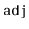, of lists. The listcontains a list of all the vertices adjacent to vertex. That is, it contains every index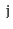such that.

    int n;
List<Integer>[] adj;
AdjacencyLists(int n0) {
n = n0;
adj = (List<Integer>[])new List[n];
for (int i = 0; i < n; i++)
adj[i] = new ArrayStack<Integer>(Integer.class);
}

(An example is shown in Figure 12.3.) In this particular implementation, we represent each list inas an ArrayStack, because we would like constant time access by position. Other options are also possible. Specifically, we could have implementedas a DLList.

Figure 12.3: A graph and its adjacency lists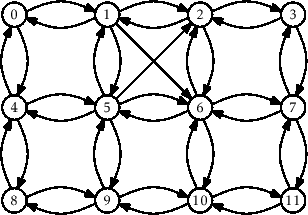0 1 2 3 4 5 6 7 8 9 10 11 1 0 1 2 0 1 5 6 4 8 9 10 4 2 3 7 5 2 2 3 9 5 6 7 6 6 8 6 7 11 10 11 5 9 10 4

The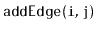operation just appends the valueto the list:

    void addEdge(int i, int j) {
adj[i].add(j);
}

This takes constant time.

The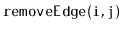operation searches through the list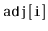until it findsand then removes it:

    void removeEdge(int i, int j) {
Iterator<Integer> it = adj[i].iterator();
while (it.hasNext()) {
if (it.next() == j) {
it.remove();
return;
}
}
}

This takestime, where(the degree of) counts the number of edges inthat have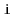as their source.

The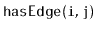operation is similar; it searches through the listuntil it finds(and returns true), or reaches the end of the list (and returns false):

    boolean hasEdge(int i, int j) {
return adj[i].contains(j);
}

This also takestime.

Theoperation is very simple; it returns the list:

    List<Integer> outEdges(int i) {
return adj[i];
}

This clearly takes constant time.

The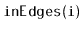operation is much more work. It scans over every vertex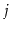checking if the edgeexists and, if so, addingto the output list:

    List<Integer> inEdges(int i) {
List<Integer> edges = new ArrayStack<Integer>(Integer.class);
for (int j = 0; j < n; j++)
if (adj[j].contains(i))    edges.add(j);
return edges;
}

This operation is very slow. It scans the adjacency list of every vertex, so it takestime.

The following theorem summarizes the performance of the above data structure:

Theorem 12..2   The AdjacencyLists data structure implements the Graph interface. An AdjacencyLists supports the operations
•in constant time per operation;
•andintime per operation;
•in constant time per operation; and
•in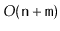time per operation.
The space used by a AdjacencyLists is.

As alluded to earlier, there are many different choices to be made when implementing a graph as an adjacency list. Some questions that come up include:

• What type of collection should be used to store each element of? One could use an array-based list, a linked-list, or even a hashtable.
• Should there be a second adjacency list,, that stores, for each, the list of vertices,, such that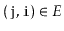? This can greatly reduce the running-time of theoperation, but requires slightly more work when adding or removing edges.
• Should the entry for the edgeinbe linked by a reference to the corresponding entry in?
• Should edges be first-class objects with their own associated data? In this way,would contain lists of edges rather than lists of vertices (integers).
Most of these questions come down to a tradeoff between complexity (and space) of implementation and performance features of the implementation.

opendatastructures.org